Essence of the Thought Experiment
 The thought experiment which the founding 1905 paper  of Einstein's theory of relativity is based on concerns a rigid rod with ends A and B, moving in the stationary system K at velocity v parallel to the x-axis (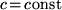is the velocity of light): "Let a ray of light depart from A at the time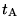, let it be reflected at B at the time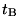, and reach A again at the time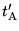. Taking into consideration the principle of the constancy of the velocity of light we find that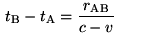(click on the formula to see derivation) and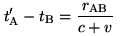(click on the formula to see derivaltion) where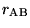denotes the length of the moving rod -- measured in the stationary system." The first of the above formulae expresses the time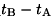necessary for the light to travel from A to B, measured by the clocks in K where end A and end B of the rigid rod happen to be in K, while the second expresses the time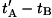necessary for the light to travel from B back to A, again, measured by the clocks placed where ends A and B happen to be in K. It cannot be emphasized stronger that the above formulae are derived by the stationary observers (observers in K) for the purposes of drawing a conclusion as to what the observers moving with the rod (observers in k) see. Since the observers in K have at their disposal only the parameters of the stationary system K, Einstein needed an additional rule which would allow the observers in K to become speakers for the observers in k: To solve the contradiction between the fact that the indications of the clocks participating in the above inequality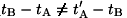(undoubtedly, therefore, an inequality derived in the stationary system K), are indications of clocks in the stationary system K, while for the purposes of his conclusion these quantities have to be indications of clocks also in the moving system k, Einstein spells out explicitly the following obvious rule for the connection between the readings of clocks in the stationary system K and the readings of the clocks in the moving system k spatially coinciding with them (rule for the synchronicity in K of spatially coinciding clocks): "We imagine further that at the two ends A and B of the rod, clocks are placed which synchronize with the clocks of the stationary system, that is to say that their indications correspond at any instant to the "time of the stationary system'' at the places where they happen to be. These clocks are therefore "synchronous in the stationary system"." Having done that, Einstein now takes advantage of the obvious, while foisting on the reader the universality of, that the clocks placed at A and B (at rest with k) would be synchronous, that is, would have common time in the moving system k if and only if the above timesandobeyed the following definition for synchronicity given in §1 of the 1905 paper: "... the "time'' required by light to travel from A to B equals the "time'' it requires to travel from B to A. " However, as follows purely formally from the above formulae, under the foisted condition for the velocity of light being alwaysalso in k, that it is not so in k. On the contrary, as is seen, what follows from the above formulaeand, provided the velocity of light is under any circumstances, the purported relationship in k is always the inequalityThis inequality, derived, as said, in the stationary system K but transferred according to the above rule for the synchronicity in K of spatially coinciding clocks into the moving system k, together within k, is, according to Einstein, the proof that the clocks at A and B in the moving system k do not have common time, that is, that: "Observers moving with the moving rod would thus find that the two clocks were not synchronous". Once again, this is so because, obviously, in order for the clocks in question to have common time when, they have to obey the following, already mentioned, evident criterion for synchronicity, given in §1 of the 1905 paper: "... the "time'' required by light to travel from A to B equals the "time'' it requires to travel from B to A. " which is a criterion clearly not obeyed by the above-observed inequalityif indeedin k. This section is devoted to explaining what the essence of the thought experiment in  is. Nevertheless, it is imperative to state at once even at this point that, of course, the above conclusion, namely, that the clocks at A and B moving with the rod are non-synchronous, made in the described way, is in error. As can clearly be seen, the very formulaeandEinstein uses to prove such non-synchronicity in fact prove conclusively that the clocks in question are, in fact, nothing but synchronous. The above erroneous conclusion for the non-synchronicity of the clocks at A and B in k can only be arrived at if one "forgets" the obvious truth that the clocks at A and B are nothing but synchronous (the latter follows directly from the very formulae Einstein presents in §2 of ), and one only decides to give credence to the joint validity of the only possible relationship between times,andin k, namely, the inequalityon the one hand and on the other the equality, pronounced to be the universal velocity of light in any system, including k. Such "forgetting" of the truth and forging imposed conditions so that it would appear that one proves one's point is clearly a completely unacceptable manipulation which has no place in any logical discourse let alone in a discourse in science. On the other hand, for, for a rigid rod at rest with the stationary system K, the valid relationship for the observers in K (observers at rest with the rod) is obviously the equality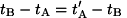, which according to the above definition is the basis to assert, as Einstein correctly does, that the clocks at A and B of the rigid rod, immovable with respect to K, do have common time and are synchronous, that is, to state that "while observers in the stationary system would declare the clocks to be synchronous." Based on the above Einstein makes the following (incorrect) general conclusion. "So we see that we cannot attach any absolute signification to the concept of simultaneity, but that two events which, viewed from a system of co-ordinates, are simultaneous, can no longer be looked upon as simultaneous events when envisaged from a system which is in motion relatively to that system." considered as one of the greatest discoveries of all time, known under the term "relativity of simultaneity", which underlies anything Einstein's theory of relativity represents. As explained, the conclusion for "relativity of simultaneity" is incorrect and that is seen directly from the very formulae presented by Einstein with the aim to prove it. One may also consider the following, slightly modified, way of debunking the claim for the "relativity of simultaneity": It is seen at once [link][link] that for a source of light at rest with the stationary system, the formulaeandwhich Einstein uses to "prove" relativity of simultaneity are derived purely classically (without Einstein's theory of relativity), despite Einstein's insistence in §2 of his 1905 paper . Thus, having these formulae present in classical physics (the physics without Einstein's theory of relativity) the only thing needed for the relativity of simultaneity to be claimed is to postulate that "light is always propagated in empty space with a definite velocity c which is independent of the state of motion of the emitting body", that is, thatunder any circumstances (this is known as the second postulate of Einstein's theory of relativity; cf. the preamble of his founding 1905 paper ). However, to postulate thatis the universal velocity of light would be tantamount to postulating that the clocks at ends A and B of the rod, moving with the moving rod, are always non-synchronous because universality ofleads to the inevitable derivation of solely the inequalityin the moving system k -- recall, joint validity of the inequalityand the equalityin a given system is impossible for synchronous clocks; only the readings of non-synchronous clocks can exhibit the inequalitywhen. However, as seen, the very formulae Einstein uses to "prove" non-synchronicity of the clocks at A and B in k directly show the opposite, namely, that these clocks are nothing but synchronous. As was said above, the discussed erroneous conclusion for "relativity of simultaneity" can only be arrived at if one "forgets" the obvious truth that the clocks at A and B are nothing but synchronous (following from the very formulae Einstein presents in §2 of ), and only decides to gives credence to the joint validity of the only possible relationship between times,andin k, namely, the inequalityon one hand and on the other the equality, pronounced to be the universal velocity of light in any system, including k. This "forgetting" of the truth and forging imposed conditions with the aim to appear that one proves one's point is clearly a completely unacceptable manipulation which has no place in any logical discourse let alone a dicourse in science. If one needs the above to be stated in a different way then one may observe that an initial condition comprising a postulate such as the one requiring universality ofis unfounded because it contradicts another initial condition -- validity of Newton's mechanics in the stationary system K. As seen Newton's mechanics inevitably leads to the fact that two synchronous clocks in one system remain synchronous in all systems. Postulating in the same breath universality ofis tantamount to postulating that the opposite is true because then, as said, the only possible relationship between times intervalsandread from the faces of clocks in k is the inequality, which means that the only possibility for the clocks in k whenis to be non-synchronous. This is a contradiction -- the clocks at A and B are always synchronous but the postulate for the universality ofderives that they are always non-synchronous. Therefore, Einstein's inferrence that the observers in the two different systems will arrive at different conclusions regarding simultaneity of one and the same pair of clocks in one and the same system k, is nothing else but a most common mistake and is contradictory in itself. It is a result of flagrant manipulation of contradictory initial conditions.